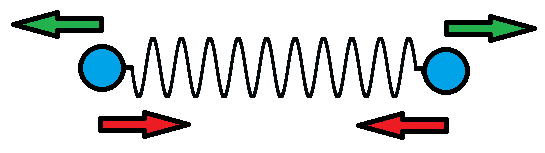# Coulomb vs HookeThere are two identical charges attached to the ends of a spring. How far apart are they if the system is in equilibrium (neglecting gravity)?

System Parameters (All in SI units):

 Charge Coulomb's constant Spring constant Spring unstretched length $q = 10^{-4} C$ $k = 9\times10^9 \text{ Nm}^2 \text{ C}^{-2}$ $\alpha = 90\text{ Nm}^{-1}$ $L = 1\text{ m}$
×

Problem Loading...

Note Loading...

Set Loading...